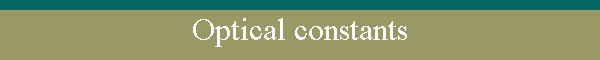- (R,T) method - compute optical constants of absorbent layers knowing (R,T) points and geometrical thickness. If the layer have non-absorbent spectral range with extremes value in R/T, the geometrical thickness can be computed.     - Envelope method - compute optical constants of weekly absorbent layers.     - Dispersion equations - compute equation coefficients of dispersion.     - Save materials - in STICLE32.DAT file.